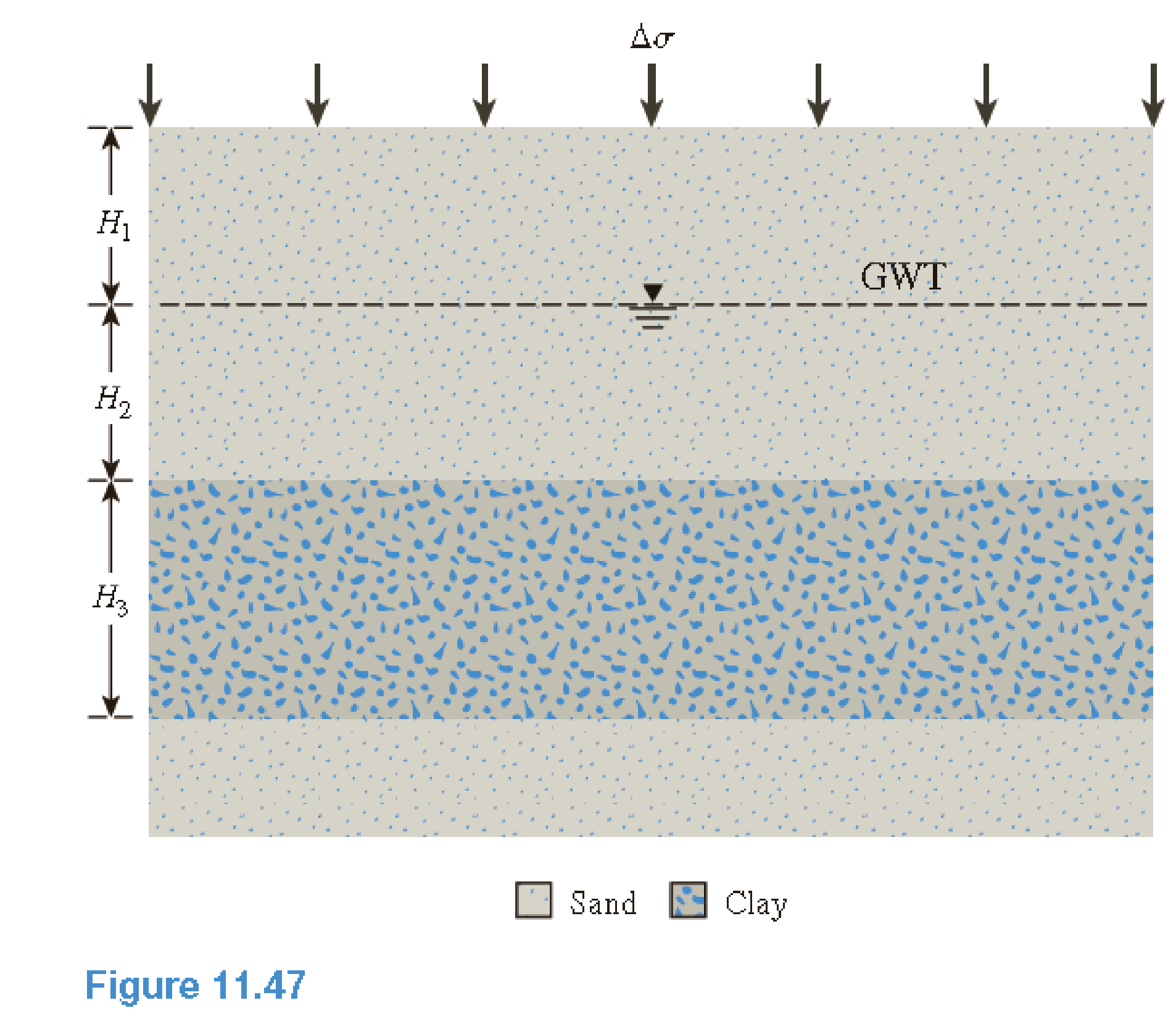Chapter 11, Problem 11.15PPrinciples of Geotechnical Enginee...

9th Edition
Braja M. Das + 1 other
ISBN: 9781305970939

Solutions

Chapter
SectionPrinciples of Geotechnical Enginee...

9th Edition
Braja M. Das + 1 other
ISBN: 9781305970939
Textbook Problem

Refer to Problem 11.11 a. What would be the degree of consolidation in the middle of the clay layer 180 days after the application of the foundation load? Given: cv = 0.18 cm2/min. b. What would be the remaining excess pore water pressure in the middle of the clay layer 180 days after construction? 11.11 Refer to Figure 11.47. Estimate the primary consolidation settlement in the clay layer. Given: Δ σ = 105   kN / m 2 , H 1 = 2.2   m , H 2 = 4.4   m , and H 3 = 7.5   m . Soil characteristics areSand: e = 0.58; Gs = 2.67Clay: LL = 49; e = 1.08; Gs = 2.71; σ ′ c = 210   kN / m 2 ; C s = 1 6 C c(a)

To determine

Calculate the degree of consolidation in the middle of the clay layer.

Explanation

Given information:

The coefficient of consolidation (cv) is 0.18cm2/min.

The height of the sand layer 1 (H1) is 2.2m.

The height of the sand layer 2 (H2) is 4.4m.

The height of the clay layer 3 (H3) is 7.5m.

The time taken for consolidation (t) is 180days.

The net applied pressure (Δσ) is 105kN/m2.

The depth (z) is 3.75m.

Calculation:

Calculate the length of maximum drainage path (Hdr) using the relation.

Hdr=H32

Substitute 7.5m for H3.

Hdr=7.52=3.75m×100cm1m=375cm

Calculate the time factor (Tv) as shown below.

Tv=cvtHdr2

Substitute 180days for t, 0.18cm2/min for cv, and 375cm for Hdr

(b)

To determine

Calculate the excess pore water pressure in the middle of the clay layer.

Still sussing out bartleby?

Check out a sample textbook solution.

See a sample solution

The Solution to Your Study Problems

Bartleby provides explanations to thousands of textbook problems written by our experts, many with advanced degrees!

Get Started

Explain the differences among data, information, and a database.

Database Systems: Design, Implementation, & Management

What is the major difference between counterboring and spotfacing?

Precision Machining Technology (MindTap Course List)

What e user applications? Suggest three examples that could boost user productivity.

Systems Analysis and Design (Shelly Cashman Series) (MindTap Course List)

What are some skills that entry-level welders need?

Welding: Principles and Applications (MindTap Course List)